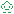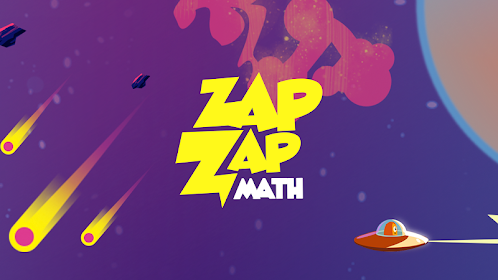# Fraction Basics - Easy & Effective Fractions TutorEveryoneAges 9-12
6
Offers in-app purchasesIf you’re struggling with fraction problems or looking for a quick, smart and effective way to master the basics, Fraction Basics is your answer. This free video app teaches you the core concepts of fractions in 12 computer-animated, visually interactive videos.

Fraction Basics is designed to help you easily navigate the videos to access just the lesson you need to tackle, and you can easily skip to your problem areas or targeted sections. Our video app is divided into the following parts listed below to help you build a strong foundation in fractions:

1. Understanding Fractions
• Introduction
• Numerator, denominator of a fraction & proper fractions
• The meaning of fractions
• Improper fractions & mixed fractions
• How improper fractions & mixed fractions are related

2. Improper & Mixed Fractions
• Introduction
• Proper fractions & improper fractions
• Convert an improper fraction to a mixed fraction
• Quicker way of converting improper fractions to mixed fractions
• Convert mixed fractions to improper fractions

3. Equivalent Fractions
• What are equivalent fractions?
• Examine equivalent fractions visually (by multiplication)
• Reasoning behind equivalent fractions
• Can we get equivalent fractions by division?
• Can we get equivalent fractions by addition or subtraction?

4. Adding Fractions
• Introduction: Quick recap on fractions
• Basics behind adding fractions with like denominators
• Basics behind adding fractions with unlike denominators
• Example on adding fractions with unlike denominators
• Convert an improper fraction to a mixed fraction after adding

5. Adding Fractions Examples
• Example 1: Adding a whole number with a proper fraction
• Quick way of adding for Example 1 above
• Example 2: Adding a mixed fraction with a proper fraction (Like Denominators)
• Example 3: Adding a mixed fraction with a proper fraction (Unlike Denominators)
• Convert an improper fraction to a mixed fraction after adding

6. Subtracting Fractions
• Introduction: The idea behind subtracting fractions
• Visually examine how the subtraction works
• Example 1: Subtracting two fractions with unlike denominators
• Example 2: Subtracting a proper fraction with a mixed fraction
• Convert an improper fraction to a mixed fraction after subtracting

7. Multiplying Fractions
• Introduction
• Example 1: Multiplying two proper fractions
• Examine how multiplying fractions work in Example 1
• Example 2: Multiplying two fractions & simplifying the result
• Example 3: Multiplying a proper fraction and a mixed fraction & simplifying the result

8. Multiplying Fractions Examples
• Introduction
• Example 1: Simplify before multiplying two proper fractions
• Example 2: More on simplifying fractions before multiplying
• Example 3: Multiplying two mixed fractions
• Simplify before multiplying for Example 3

9. Dividing Fractions
• Introduction
• Example 1: Dividing two proper fractions
• Examine the ideas behind dividing fractions in Example 1
• Example 2: Another example on dividing fractions
• Further simplifying the expression in Example 2

10. Dividing Fractions Examples
• Example 1: Dividing two proper fractions
• Example 2: Dividing a proper fraction with a mixed fraction
• Converting the mixed fraction to an improper fraction before dividing
• Example 3: Dividing two mixed fractions
• Further simplifying the expression in Example 3

11. Convert Fraction to Percent
• Introduction
• Converting two fifths to percent
• Examine the percentage when the numerator is increased
• A quicker way of converting two fifths to percent
• Converting three fourths to percent

12. Convert Decimal to Fraction
• Introduction
• Analyze the fraction, one half
• Example 1: Convert 0.5 to a fraction
• Example 2: Convert 0.19 to a fraction
• Example 3: Convert 0.128 to a fraction
Read more
Collapse

Review Policy
2.7
6 total
5
4
3
2
1
Loading...

## What's New

- Added option to sign in with Google account.
Read more
Collapse

## Additional Information

Updated
May 16, 2019
Size
56M
Installs
1,000+
Current Version
3.1.3
Requires Android
4.1 and up
Content Rating
Everyone
Interactive Elements
Digital Purchases
In-app Products
\$1.99 - \$18.99 per item
Permissions
Offered By
Visual Math Interactive
Developer
D-67-3A, Block D, Level 3A, Jaya One, No. 72A Jalan Universiti, Petaling Jaya, Selangor, Malaysia.©2019 GoogleSite Terms of ServicePrivacyDevelopersArtistsAbout Google|Location: United StatesLanguage: English (United States)
By purchasing this item, you are transacting with Google Payments and agreeing to the Google Payments Terms of Service and Privacy Notice.